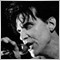# Price2041

Is there any way of locking the price value to a given point in time, i know that you can display a set price for the Close of a candle for example however i want to display the price at the point that an Alert is given for say the crossover of 2 MA's just like the read out you can get with the pop up Alert box, all i get at present is a price when the signal is given but the price then proceeds to update with the current price and i cannot seem to lock it to the actual signal time. Any help or suggestions would be most appreciated.

cja157

cja:
Is there any way of locking the price value to a given point in time, i know that you can display a set price for the Close of a candle for example however i want to display the price at the point that an Alert is given for say the crossover of 2 MA's just like the read out you can get with the pop up Alert box, all i get at present is a price when the signal is given but the price then proceeds to update with the current price and i cannot seem to lock it to the actual signal time. Any help or suggestions would be most appreciated. cja

cja,

Why not just store the price (at the time of crosssing) in a variable and use that variable to display/pop-up/print etc? (It sounds simple. May be I did not understand your question properly).

Thx.2041

Setting price

fxd01:
cja,

Why not just store the price (at the time of crosssing) in a variable and use that variable to display/pop-up/print etc? (It sounds simple. May be I did not understand your question properly).

Thx.

Can you give me an example of what you are talking about, and i will see if i can apply it to my situation, thanks157

cja:
Can you give me an example of what you are talking about, and i will see if i can apply it to my situation, thanks

cja,

If I understood your question correctly, this may work (otherwise my appologies).

1. define couple of variales before the start() function. Note that the crossPrice variable is created as static variable so that the value assigned to remains static until changed

static double crossPrice;

int crossDirection; //1=up, 2=down, 0=no direction

2. check the crossing of the MA's (I just used a period of 6 & 13). If the MA's crossed, assign the price (it could be ask, bid or (ask+bid/2).

crossDirection=0;

double ma_06_0=iMA(NULL,0,6,0,MODE_SMA,PRICE_CLOSE,0);

double ma_13_0=iMA(NULL,0,13,0,MODE_SMA,PRICE_CLOSE,0);

double ma_06_1=iMA(NULL,0,6,0,MODE_SMA,PRICE_CLOSE,1);

double ma_13_1=iMA(NULL,0,13,0,MODE_SMA,PRICE_CLOSE,1);

if (ma_06_0>ma_13_0 && ma_06_1<ma_13_1)

{

crossDirection=1; // ie. direction is up

}

if (ma_13_0>ma_06_0 && ma_13_1<ma_06_1)

{

crossPrice=Bid; // or bid or (ask+bid)/2

crossDirection=2; // ie. direction is down

}

The Value in crossPrice remains unchanged until the next cross occurs. Please let me know if this is what you are looking for.2041

Static Price

fxd01:
cja,

If I understood your question correctly, this may work (otherwise my appologies).

1. define couple of variales before the start() function. Note that the crossPrice variable is created as static variable so that the value assigned to remains static until changed

static double crossPrice;

int crossDirection; //1=up, 2=down, 0=no direction

2. check the crossing of the MA's (I just used a period of 6 & 13). If the MA's crossed, assign the price (it could be ask, bid or (ask+bid/2).

crossDirection=0;

double ma_06_0=iMA(NULL,0,6,0,MODE_SMA,PRICE_CLOSE,0);

double ma_13_0=iMA(NULL,0,13,0,MODE_SMA,PRICE_CLOSE,0);

double ma_06_1=iMA(NULL,0,6,0,MODE_SMA,PRICE_CLOSE,1);

double ma_13_1=iMA(NULL,0,13,0,MODE_SMA,PRICE_CLOSE,1);

if (ma_06_0>ma_13_0 && ma_06_1<ma_13_1)

{

crossDirection=1; // ie. direction is up

}

if (ma_13_0>ma_06_0 && ma_13_1<ma_06_1)

{

crossPrice=Bid; // or bid or (ask+bid)/2

crossDirection=2; // ie. direction is down

}

The Value in crossPrice remains unchanged until the next cross occurs. Please let me know if this is what you are looking for.

Thanks for your time to explain this i will try it out later tonight and let you know how it goes.

cja157

cja:
Thanks for your time to explain this i will try it out later tonight and let you know how it goes. cja

No problem cja. I will be happy if I am of any help. I have seen many wonderful indicators created by you.2041

Static price

fxd01:
No problem cja. I will be happy if I am of any help. I have seen many wonderful indicators created by you.

Thanks for the code - i applied it to my price signal and it certainly works as far as locking the price at the signal time but if you change timeframes the price updates - any ideas?? i will have more of a look into it tonight.

Thanks for your help once more

cja401

Why not write it to an external log file along with time, date and any other relevant variables?

Lux